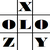anything in Excel...

It's Excel-o-lozy...

### Excel formula to convert Number to Text in formulaFix by Excelolozy

www.excelolozy.com - To convert Number, values or digits to Text is easy through "Num2txt" formula.

This formula converts any number or digit as follows :
• e.g. to convert 2,735 to text following is the solution:
=Num2txt(2735) result of which is shown as "Two Thousand Seven Hundred Thirty Five".

More over this formula has also option of showing prefix and suffix to the resulting text.

• e.g. to prefix "INR" to above example:=Num2txt(2735,"INR") result of which is shown as "INR Two Thousand Seven Hundred Thirty Five"
• e.g. to suffix "only" to above example:=Num2txt(2735,"INR","only") result of which is shown as "INR Two Thousand Seven Hundred Thirty Five only"
The formula "Num2Txt" is available within tool named "formulaFix".

"formulaFix" is a "Formula Fixing Tool" that provides various formulas that dont exist in Excel.